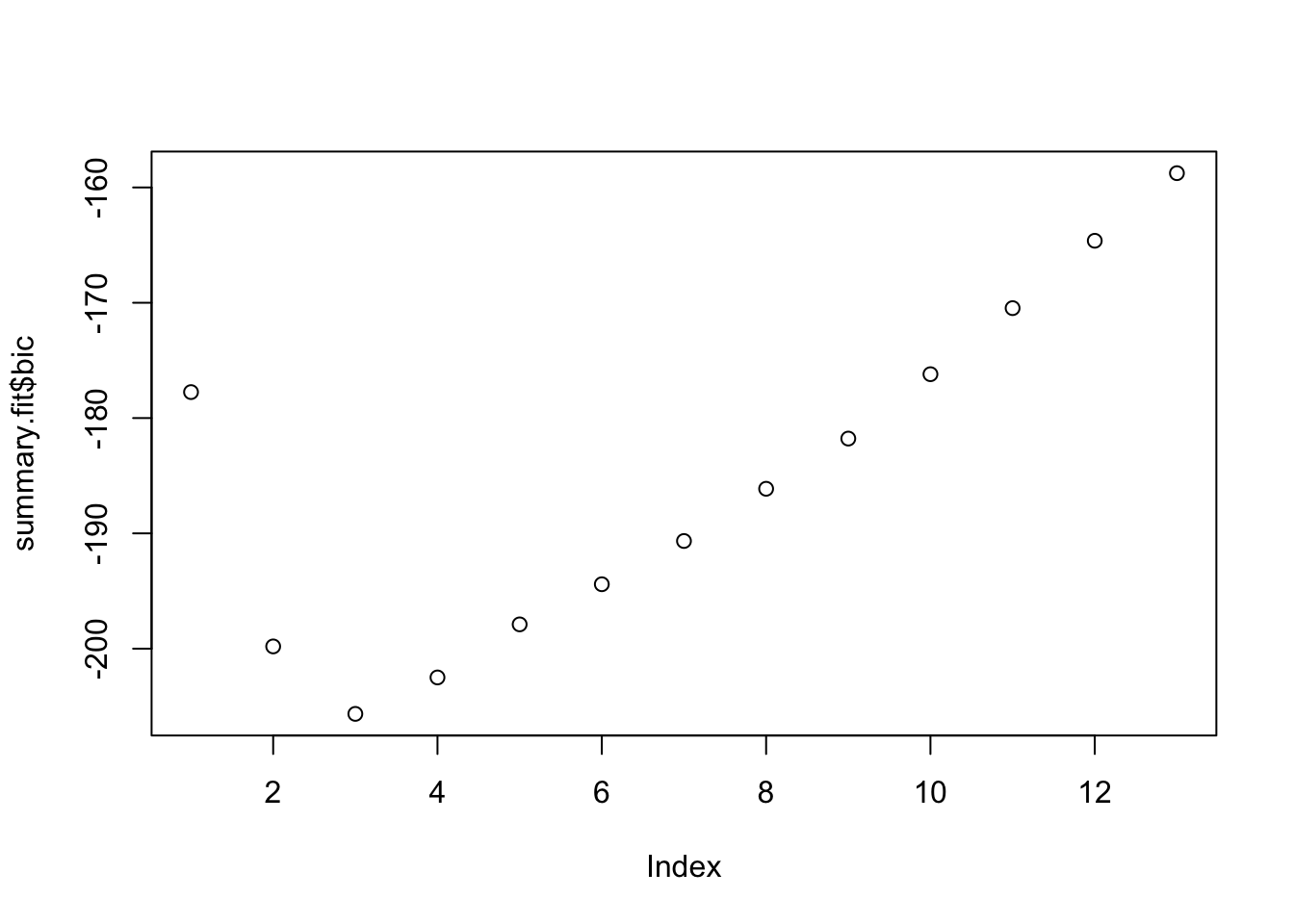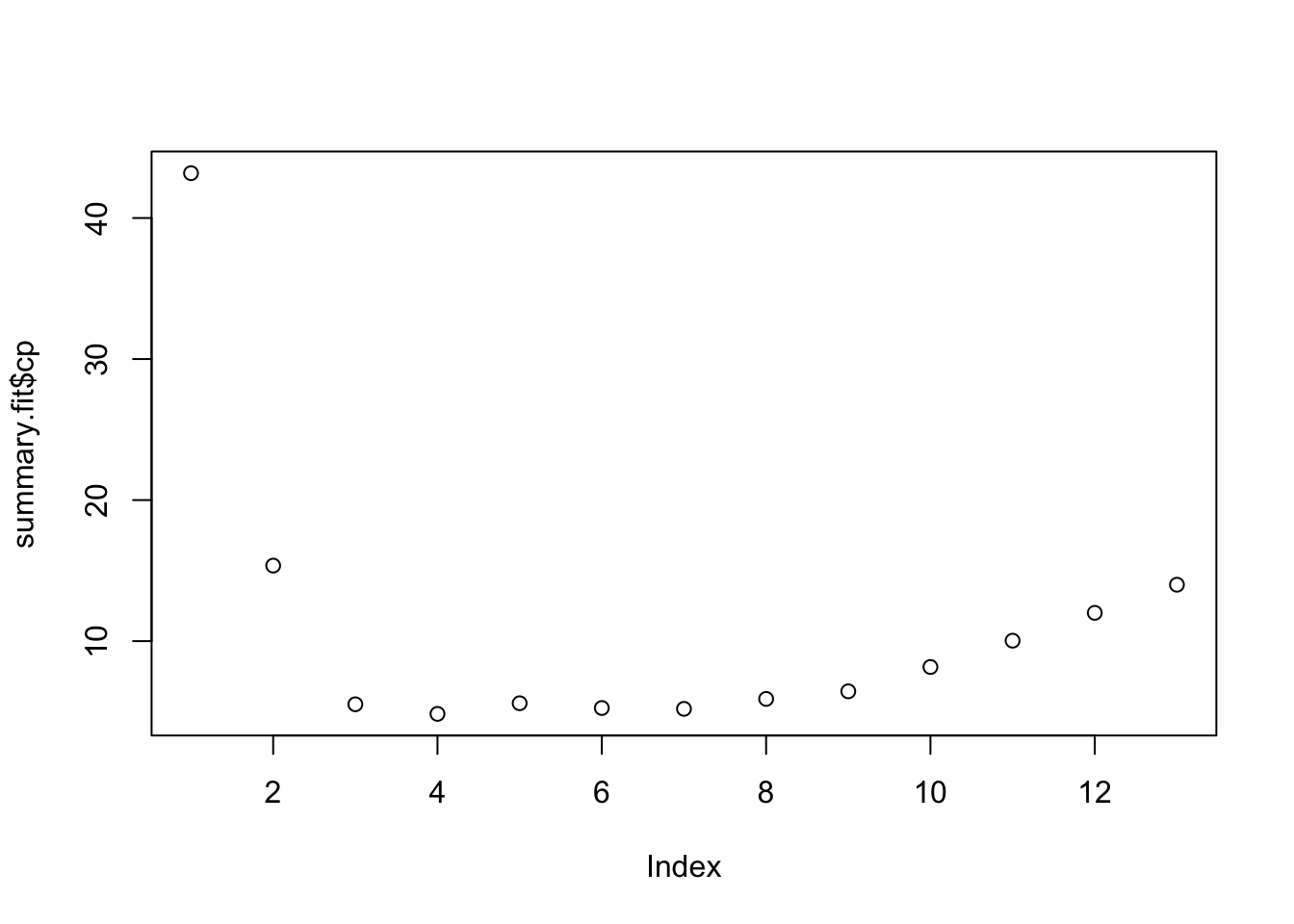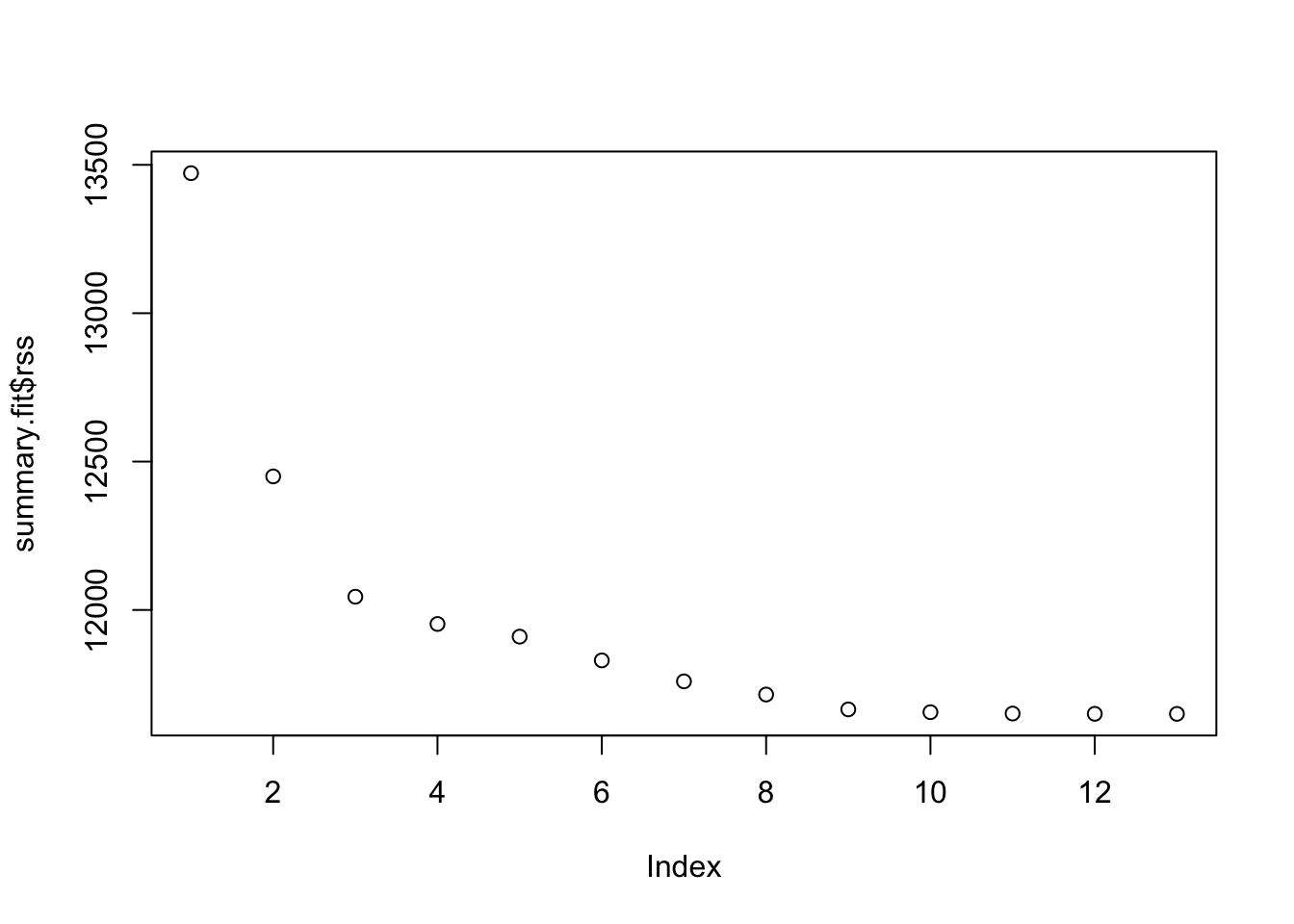ISLR Home

# Question

p264

We will now try to predict per capita crime rate in the Boston data set.

1. Try out some of the regression methods explored in this chapter, such as best subset selection, the lasso, ridge regression, and PCR. Present and discuss results for the approaches that you consider.

2. Propose a model (or set of models) that seem to perform well on this data set, and justify your answer. Make sure that you are evaluating model performance using validation set error, cross- validation, or some other reasonable alternative, as opposed to using training error.

3. Does your chosen model involve all of the features in the data set? Why or why not?

``````library(MASS)
library(leaps)``````

# 11a best subset selection

``````set.seed(1)

n = nrow(Boston)
dim(Boston)-1``````
``##  13``
``````train = sample(1:n, n*.7)
#test = (-train)
bss.model <- regsubsets(crim ~ ., data = Boston[train,], method = "exhaustive", nvmax = dim(Boston)-1)
summary.fit = summary(bss.model)

plot(summary.fit\$bic) # lower is better````````plot(summary.fit\$cp) # lower is better````plot(summary.fit\$rss) # lower is better````plot(summary.fit\$adjr2) # lower is better``